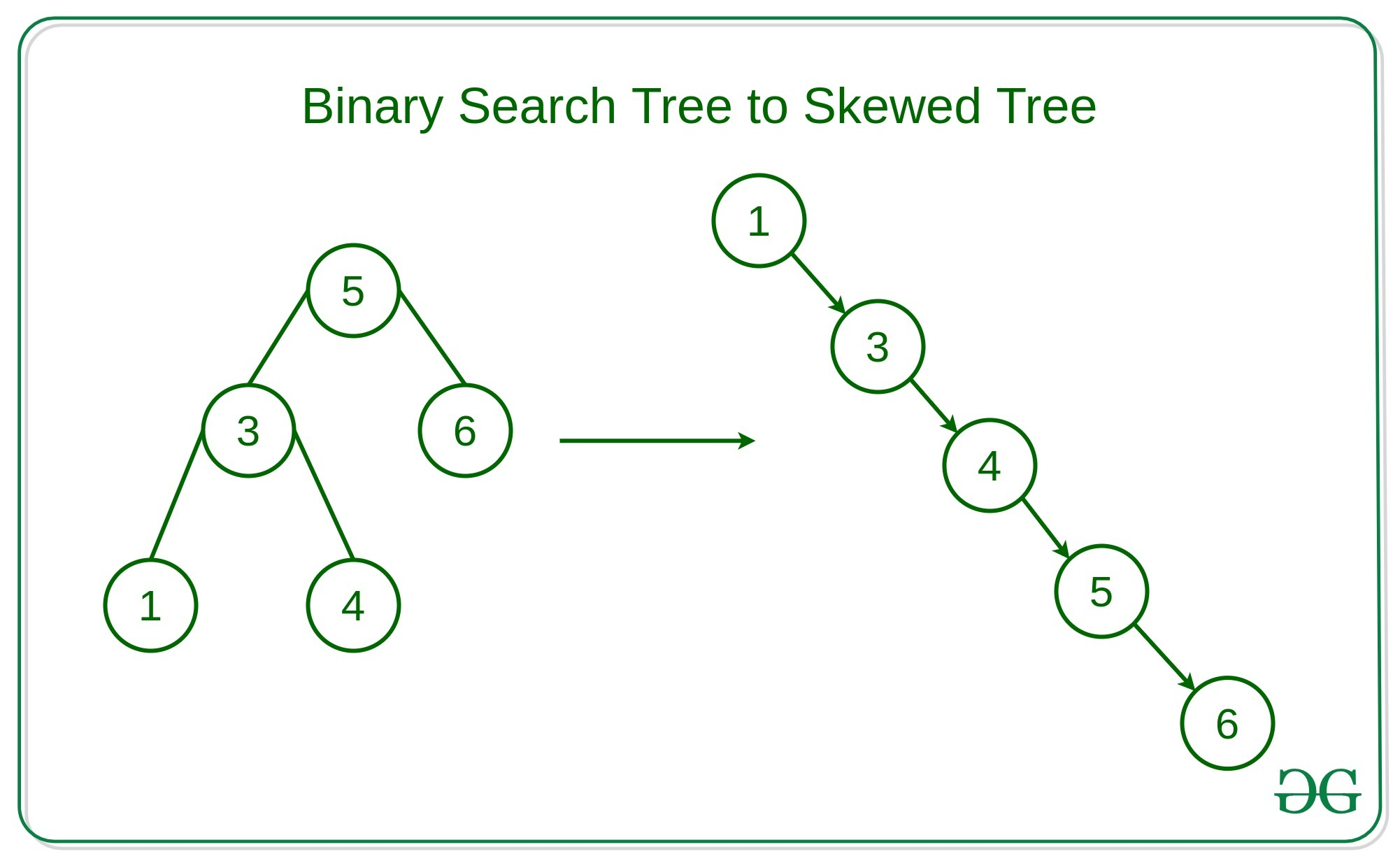# Convert a Binary Search Tree into a Skewed tree in increasing or decreasing order

Given a Binary Search Tree and a binary integer K, the task is to convert Binary search tree into a Skewed Tree in increasing order if K = 0 or in decreasing order if K = 1.Examples:

```Input: K = 0,
5
/ \
3   6
Output:
3
\
5
\
6

Input: K = 1,
2
/ \
1   3
Output:
3
\
2
\
1
```

Approach:

• The key observation in the problem is that the first node of the skewed tree will be the extreme left or extreme right node of the BST for increasing order and decreasing order respectively.
• For Increasing Order we need to do the Inorder Traversal, as the inorder traversal of a BST provides us the increasing sequence of the node values. Hence, the order of traversal at every node will be:

1. Left node: Recurse to its left node if it exists, to get smaller value.
2. Root node: After the complete traversal of its left node/subtree, connect the previous node of the skewed tree to the root node.
3. Right node: Recurse to the right node if it exists, for larger values.
• For Decreasing Order, the order of traversal at every node will be:
1. Right node: Recurse to its right node if it exists, to get larger values.
2. Root node: After the complete traversal of its right node/subtree, connect the previous node of the skewed tree to the root node.
3. Left node: Recurse to the left node/subtree for smaller values.
• Similarly, by keeping track of the previous node we can traverse the Binary search tree according to the order needed and form the skewed tree.

Below is the implementation of the above approach:

## Python

 `# Python implementation to flatten  ` `# the binary search tree into a skewed ` `# tree in increasing / decreasing order ` ` `  `# Class of the node ` `class` `Node: ` `     `  `    ``# Constructor of node ` `    ``def` `__init__(``self``, val): ` `        ``self``.val ``=` `val ` `        ``self``.left ``=` `None` `        ``self``.right ``=` `None` `         `  `prevNode ``=` `None` `headNode ``=` `None` ` `  `# Function to to flatten  ` `# the binary search tree into a skewed ` `# tree in increasing / decreasing order ` `def` `flattenBTToSkewed(root, order): ` `     `  `    ``# Base Case ` `    ``if` `not` `root: ` `        ``return` `     `  `    ``# Condition to check the order  ` `    ``# in which the skewed tree to maintained ` `    ``if` `order: ` `        ``flattenBTToSkewed(root.right, order) ` `    ``else``: ` `        ``flattenBTToSkewed(root.left, order) ` `         `  `    ``global` `headNode; ``global` `prevNode ` `    ``rightNode ``=` `root.right ` `    ``leftNode ``=` `root.left ` `     `  `    ``# Condition to check if the root Node ` `    ``# of the skewed tree is not defined ` `    ``if` `not` `headNode: ` `        ``headNode ``=` `root ` `        ``root.left ``=` `None` `        ``prevNode ``=` `root ` `    ``else``: ` `        ``prevNode.right ``=` `root ` `        ``root.left ``=` `None` `        ``prevNode ``=` `root ` `     `  `    ``# Similarly recurse for the left / right  ` `    ``# subtree on the basis of the order required ` `    ``if` `order: ` `        ``flattenBTToSkewed(leftNode, order) ` `    ``else``: ` `        ``flattenBTToSkewed(rightNode, order) ` ` `  `# Function to traverse the right ` `# skewed tree using recursion ` `def` `traverseRightSkewed(root): ` `    ``if` `not` `root: ` `        ``return` `    ``print``(root.val, end ``=` `" "``) ` `    ``traverseRightSkewed(root.right) ` ` `  `# Driver Code ` `if` `__name__ ``=``=` `"__main__"``: ` `    ``# 5 ` `    ``#      / \ ` `    ``# 3   6 ` `    ``root ``=` `Node(``5``) ` `    ``root.left ``=` `Node(``3``) ` `    ``root.right ``=` `Node(``6``) ` `     `  `    ``prevNode ``=` `None` `    ``headNode ``=` `None` `     `  `    ``# Order of the Skewed tree can ` `    ``# be defined as follows -  ` `    ``# For Increasing order - 0 ` `    ``# For Decreasing order - 1 ` `    ``order ``=` `0` `     `  `    ``flattenBTToSkewed(root, order) ` `     `  `    ``traverseRightSkewed(headNode) `

Output:

```3 5 6
```

Attention reader! Don’t stop learning now. Get hold of all the important DSA concepts with the DSA Self Paced Course at a student-friendly price and become industry ready.

My Personal Notes arrow_drop_upCheck out this Author's contributed articles.

If you like GeeksforGeeks and would like to contribute, you can also write an article using contribute.geeksforgeeks.org or mail your article to contribute@geeksforgeeks.org. See your article appearing on the GeeksforGeeks main page and help other Geeks.

Please Improve this article if you find anything incorrect by clicking on the "Improve Article" button below.